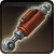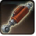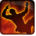# Personal VehicleConditions

## Related effects, buffs and debuffs

Please click on an effect below to view its details.

• [hidden] [does not expire]Effect #1
Movement speed increased by 90%

 Slot: Buff Duration: passive Tick rate: does not tick # occurrences: 0 Tags: tag.​abl.​player.​mount
• On Apply

Only when the following conditions are met:

• Unknown (85)
- Negated = (bool) false
- Actor = (int) 2
- Conditional = (int) 16141007604157774452

Perform the following actions:

• Set movement speed to 190%
• Custom
- Param 4 = (string)
- Param 5 = (string)
- Param 6 = (string)
- Param 7 = (string)
- Param 8 = (string)
- Param 9 = (string)
- Param 1 = (int) 9399188432865711031
- Param 3 = (int) 0
- Param 2 = (float) 70
• On Apply

Only when the following conditions are met:

• Unknown (85)
- Negated = (bool) false
- Actor = (int) 2
- Conditional = (int) 16141030317364994351

Perform the following actions:

• Set movement speed to 200%
• Custom
- Param 4 = (string)
- Param 5 = (string)
- Param 6 = (string)
- Param 7 = (string)
- Param 8 = (string)
- Param 9 = (string)
- Param 1 = (int) 9399188432865711031
- Param 3 = (int) 0
- Param 2 = (float) 70
• On Apply

Only when the following conditions are met:

• Unknown (85)
- Negated = (bool) false
- Actor = (int) 2
- Conditional = (int) 16141033615899860054

Perform the following actions:

• Set movement speed to 205%
• Custom
- Param 4 = (string)
- Param 5 = (string)
- Param 6 = (string)
- Param 7 = (string)
- Param 8 = (string)
- Param 9 = (string)
- Param 1 = (int) 9399188432865711031
- Param 3 = (int) 0
- Param 2 = (float) 70
• On Apply

Only when the following conditions are met:

• Unknown (85)
- Negated = (bool) false
- Actor = (int) 2
- Conditional = (int) 16141032516388232577

Perform the following actions:

• Set movement speed to 210%
• Custom
- Param 4 = (string)
- Param 5 = (string)
- Param 6 = (string)
- Param 7 = (string)
- Param 8 = (string)
- Param 9 = (string)
- Param 1 = (int) 9399188432865711031
- Param 3 = (int) 0
- Param 2 = (float) 70
• When NPC with this effect deals damage (weapon mode 1)

Only when the following conditions are met:

• If TARGET is in combat

Perform the following actions:

• Remove this effect
• When NPC with this effect takes damage (weapon mode 1)

Perform the following actions:

• Play appearance epp . player . mount . bike_wreck, dependent on calling effect
• Add effect #2 to TARGET from TARGET
• Remove this effect
• Just before this effect is removed

Perform the following actions:

• Custom
- Param 4 = (string)
- Param 5 = (string)
- Param 6 = (string)
- Param 7 = (string)
- Param 8 = (string)
- Param 9 = (string)
- Param 1 = (int) 9399188432865711031
- Param 3 = (int) 0
- Param 2 = (float) 50
• Dispel all effects
• [1s]Knockdown
Prone and unable to act.

 Slot: Debuff Duration: 1s Tick rate: does not tick # occurrences: 1 Tags: tag.​abl.​debuff.​knockdown Conditions:
• On Apply

Perform the following actions:

• Stun the TARGET
• Ballistic Impulse, centered at caster, immediately knockdown, momentum = 0.5, 90° pitch, 0° yaw